Ellipse - Defination

Chapter 10 Class 11 Conic Sections
Concept wise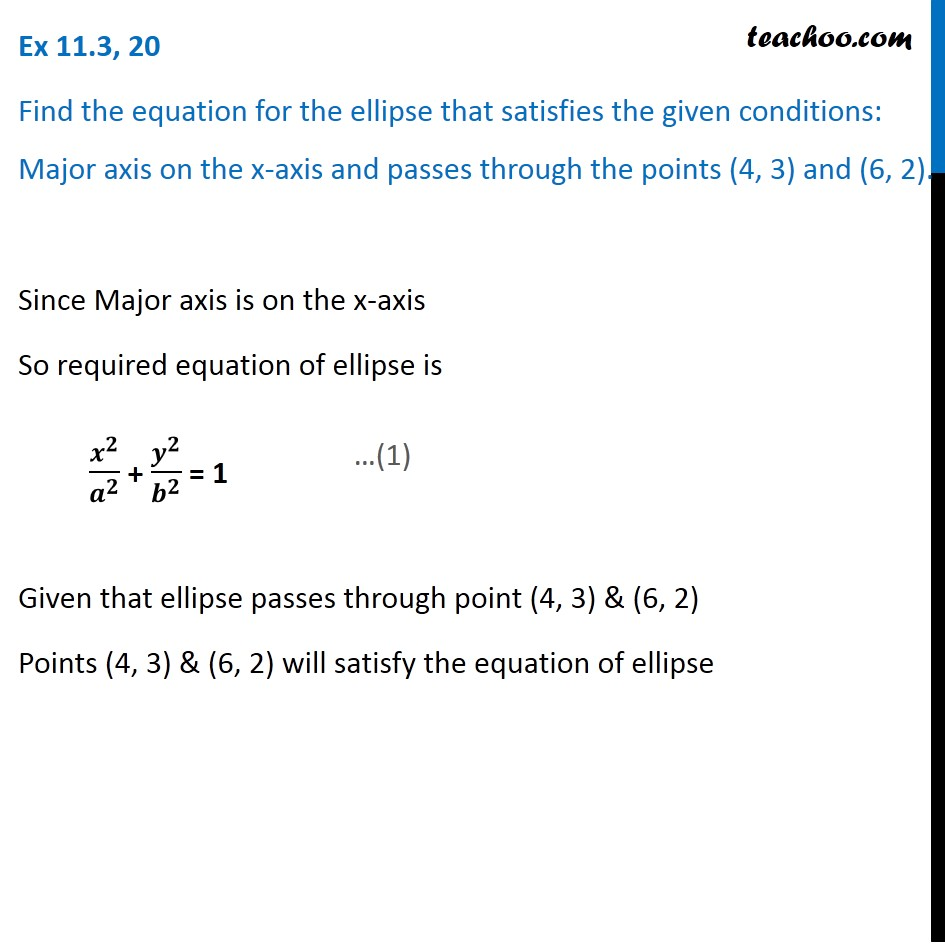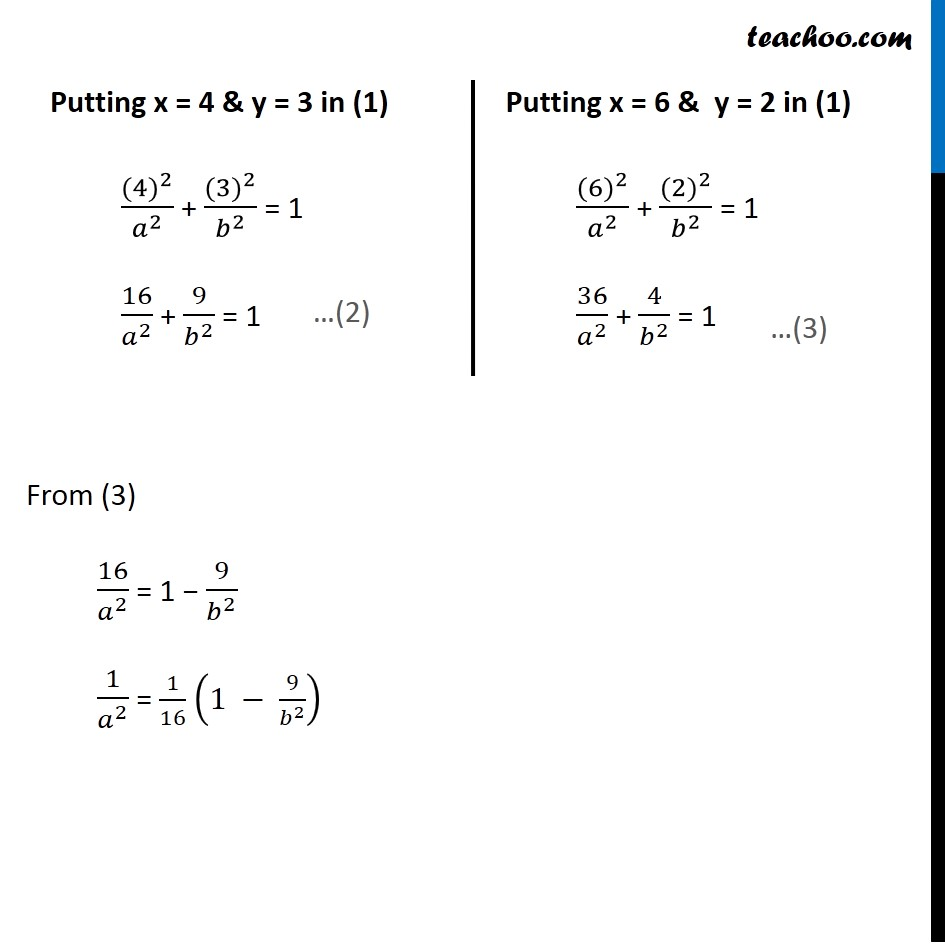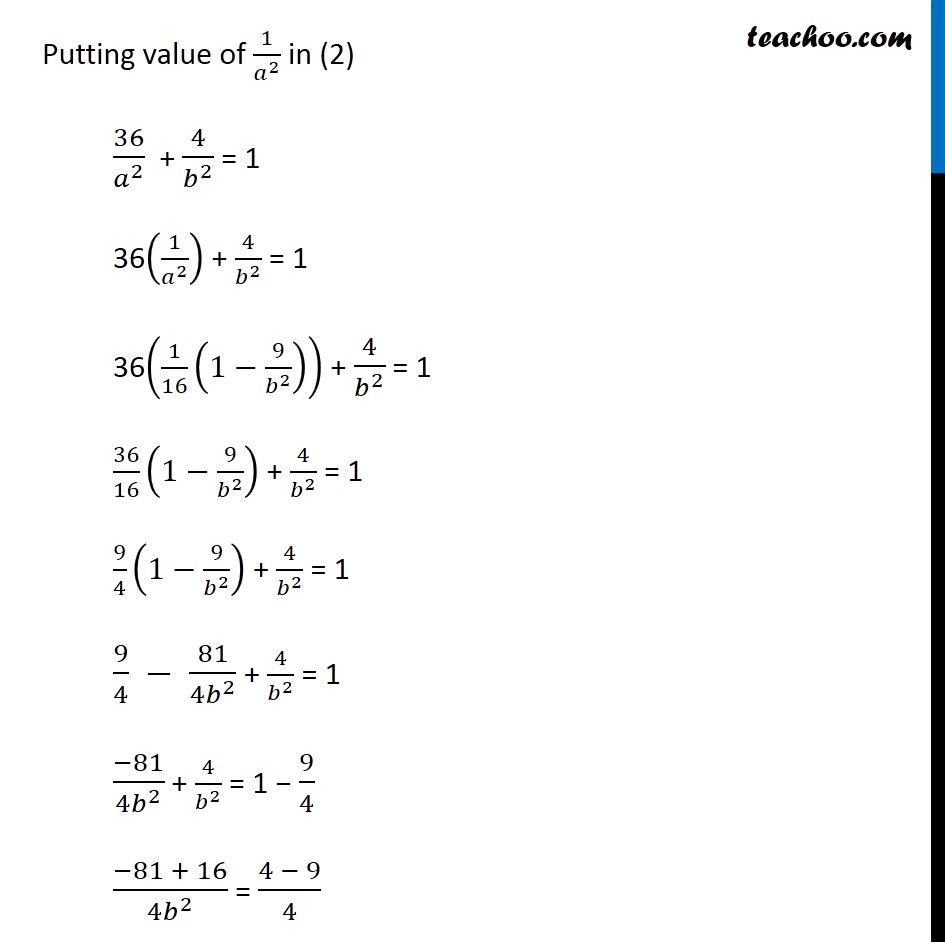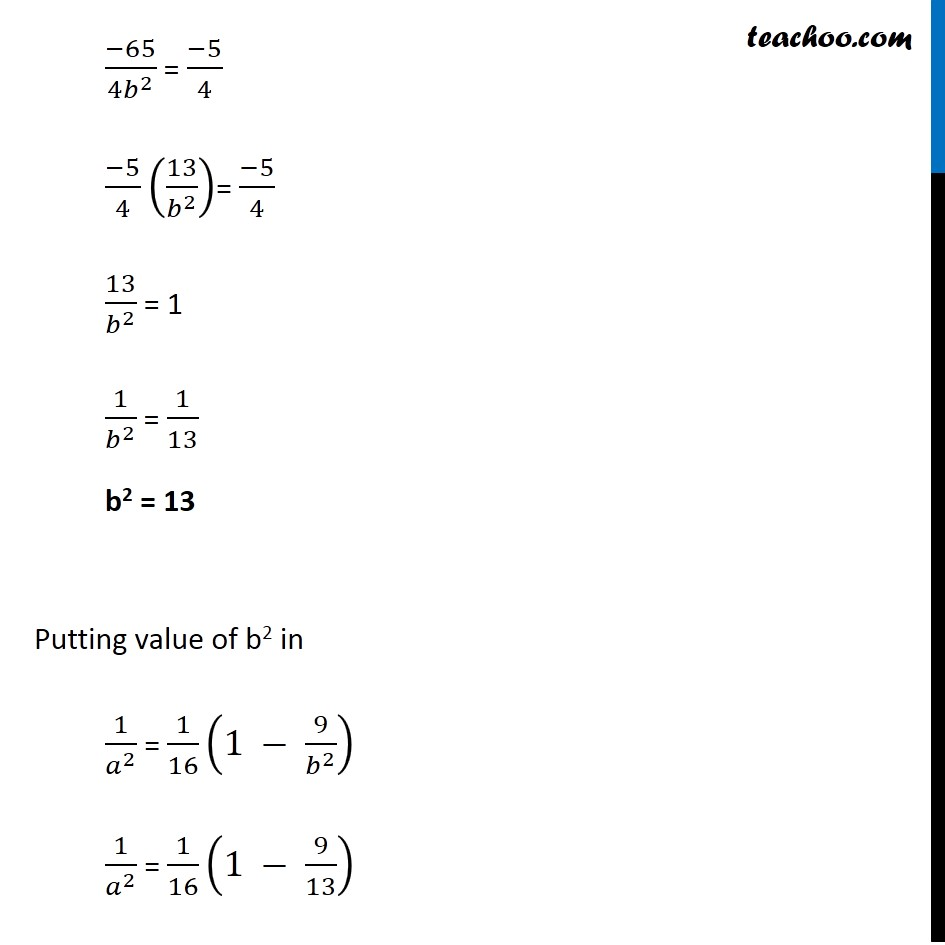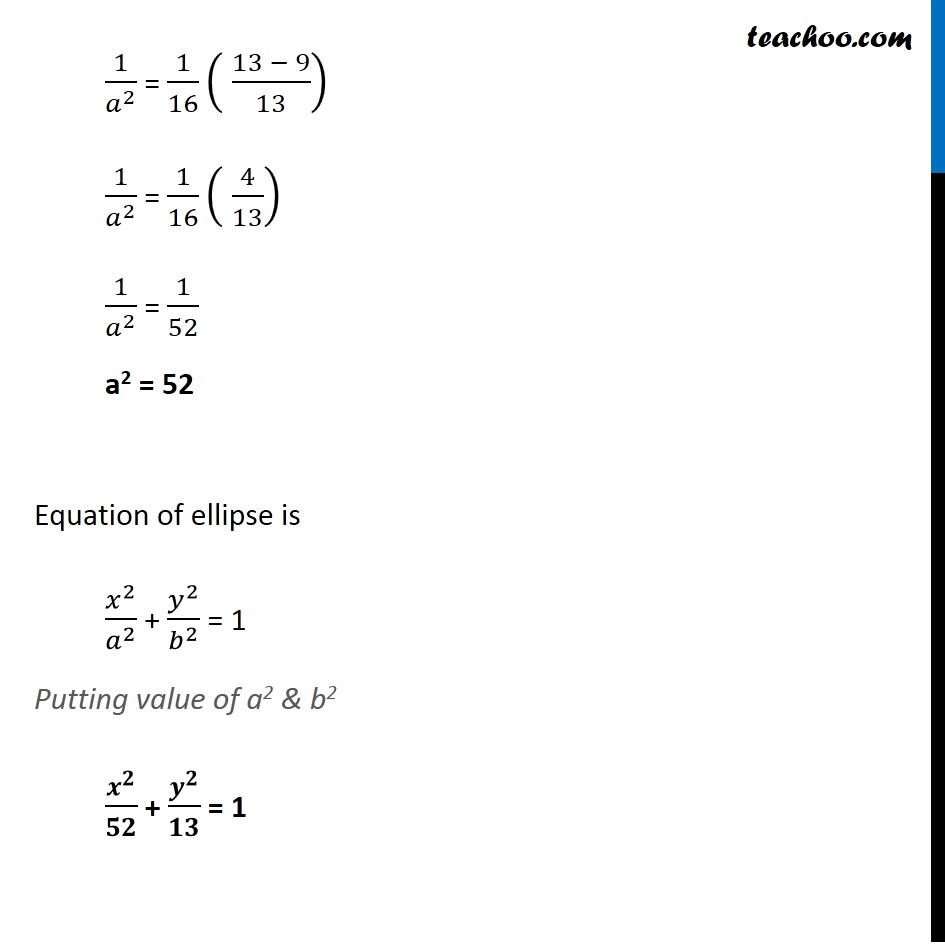Learn in your speed, with individual attention - Teachoo Maths 1-on-1 Class

### Transcript

Ex 10.3, 20 Find the equation for the ellipse that satisfies the given conditions: Major axis on the x-axis and passes through the points (4, 3) and (6, 2). Since Major axis is on the x-axis So required equation of ellipse is 𝒙^𝟐/𝒂^𝟐 + 𝒚^𝟐/𝒃^𝟐 = 1 Given that ellipse passes through point (4, 3) & (6, 2) Points (4, 3) & (6, 2) will satisfy the equation of ellipse Putting x = 4 & y = 3 in (1) 〖(4)〗^2/𝑎^2 + 〖(3)〗^2/𝑏^2 = 1 16/𝑎^2 + 9/𝑏^2 = 1 Putting x = 6 & y = 2 in (1) 〖(6)〗^2/𝑎^2 + 〖(2)〗^2/𝑏^2 = 1 36/𝑎^2 + 4/𝑏^2 = 1 From (3) 16/𝑎^2 = 1 − 9/𝑏^2 1/𝑎^2 = 1/16 (1 − 9/𝑏^2 ) Putting value of 1/𝑎^2 in (2) 36/𝑎^2 + 4/𝑏^2 = 1 36(1/𝑎^2 ) + 4/𝑏^2 = 1 36(1/16 (1−9/𝑏^2 )) + 4/𝑏^2 = 1 36/16 (1−9/𝑏^2 ) + 4/𝑏^2 = 1 9/4 (1−9/𝑏^2 ) + 4/𝑏^2 = 1 9/4 − 81/〖4𝑏〗^2 + 4/𝑏^2 = 1 (−81)/(4𝑏^2 ) + 4/𝑏^2 = 1 − 9/4 (−81 + 16)/(4𝑏^2 ) = (4 − 9)/4 (−65)/(4𝑏^2 ) = (−5)/4 (−5)/4 (13/𝑏^2 )= (−5)/4 13/𝑏^2 = 1 1/𝑏^2 = 1/13 b2 = 13 Putting value of b2 in 1/𝑎^2 = 1/16 (1 − 9/𝑏^2 ) 1/𝑎^2 = 1/16 (1 − 9/13) 1/𝑎^2 = 1/16 ( (13 − 9)/13) 1/𝑎^2 = 1/16 ( 4/13) 1/𝑎^2 = 1/52a a2 = 52 Equation of ellipse is 𝑥^2/𝑎^2 + 𝑦^2/𝑏^2 = 1 Putting value of a2 & b2 𝒙^𝟐/𝟓𝟐 + 𝒚^𝟐/𝟏𝟑 = 1